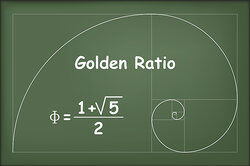# Examples of Irrational Numbers

An irrational number cannot be expressed as a ratio between two numbers and it cannot be written as a simple fraction because there is not a finite number of numbers when written as a decimal. Instead, the numbers in the decimal would go on forever, without repeating.Examples of Irrational Numbers